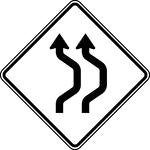Double Reverse Curve, Black and White

The Double Reverse Curve sign may be used when the tangent distance between two reverse curves is less…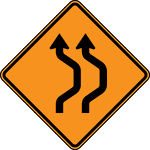Double Reverse Curve, Color

The Double Reverse Curve sign may be used when the tangent distance between two reverse curves is less…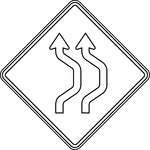Double Reverse Curve, Outline

The Double Reverse Curve sign may be used when the tangent distance between two reverse curves is less…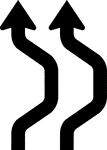Double Reverse Curve, Silhouette

The Double Reverse Curve sign may be used when the tangent distance between two reverse curves is less…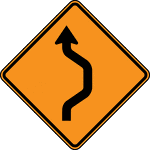Single Reverse Curve, Color

The Double Reverse Curve sign may be used when the tangent distance between two reverse curves is less…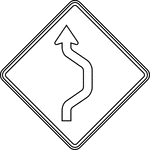Single Reverse Curve, Outline

The Double Reverse Curve sign may be used when the tangent distance between two reverse curves is less…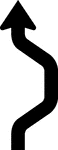Single Reverse Curve, Silhouette

The Double Reverse Curve sign may be used when the tangent distance between two reverse curves is less…Single Reverse Curve, Black and White

The Double Reverse Curve sign may be used when the tangent distance between two reverse curves is less…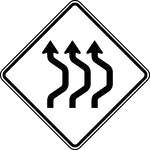Triple Reverse Curve, Black and White

The Double Reverse Curve sign may be used when the tangent distance between two reverse curves is less…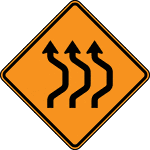Triple Reverse Curve, Color

The Double Reverse Curve sign may be used when the tangent distance between two reverse curves is less…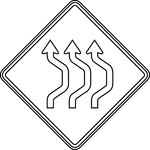Triple Reverse Curve, Outline

The Double Reverse Curve sign may be used when the tangent distance between two reverse curves is less…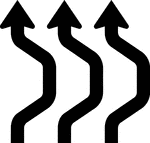Triple Reverse Curve, Silhouette

The Double Reverse Curve sign may be used when the tangent distance between two reverse curves is less…